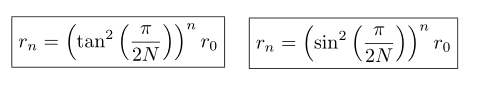# Polygon Circle FractalThe Trigonometric Fractals (a.k.a the Polygon Circle Fractals) arise when you place a regular polygon (triangle, square, pentagon, ...) either inside or around a circle. Any time you do this, it leaves a space between the central shape and the border, in which you can repeat the pattern. This describes how you build the fractals above. And while these look pretty, the question arises,

How big is each successive circle?

More specifically, what is the radius of the n'th circle? I started this problem in grade 11 or so, just for the square, and I was unable to solve it. Even after stacks and stacks of paper I never figured out how to solve this problem. After solving it ~5 years later, I know what I was missing!

The main idea to solving this (and probably most fractals) is to discover the relationship between similar shapes. For example, if you're solving the triangle inscribing a circle (left above), you can imagine drawing another triangle in any of the corners that touches the big circle. Then you have to play the geometry game and figure out how to relate an aspect of the two triangles (like the height). Once you do this, you can repeat the process indefinitely and repeat, giving you the general equations. This idea of a recurrence relation, was the missing piece. The answers below for the inscribed (left) and exscribed (right) circles are given the circle number n, and the polygon having N sides.You might notice there there are two out of the three basic trigonometric functions, tan, and sin. This suggests that there is a third! But I haven't been able to find the picture for the cos-fractal. Maybe you could give it a try!

As with most problems my first solution was very long, but after knowing the answer I could look for the reasoning for the simplified expression. Turning a multi-page proof into half a page. Along with the Mathematical Solution, I decided to write a Java Program that could generate these fractals to verify my answer. Here is a video of the program,

.
.